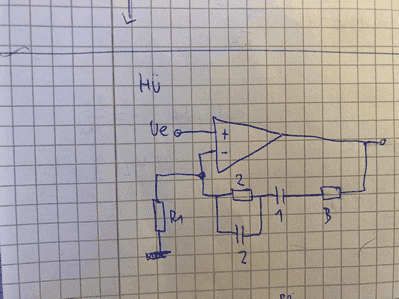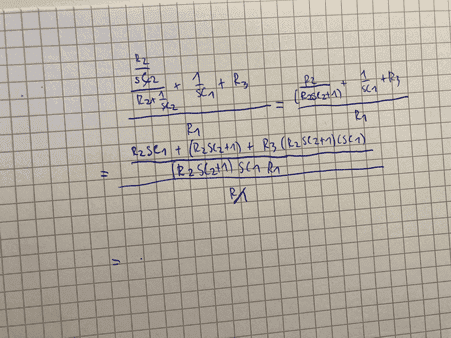# OPamp circuit - how to simplify this term?

• Engineering
• altruan23
In summary, the conversation discusses the calculation of Uout/Uin in a circuit. The denominator is in a factored form and the numerator needs to be expanded and collected into a polynomial. The quadratic terms in s cannot be factored with real numbers, so they are left in a format like (as^2 + bs + 1). The roots of the quadratic are real when Q is less than or equal to 1/2 and the quadratic is factorable with real numbers. However, since the analysis is done using variables, the general solution is not factorable and is left as a quadratic. f

#### altruan23

Homework Statement
Calculate Uout/Uin
Relevant Equations
Basic op amp eq.
So this is the circuit.And here i tried to calculate Uout/ Uin , any suggestion how to simplify this term?? I used Uout= Uin * (1+ Z2/Z1)#### Attachments

It looks good so far. The denominator is done; it's in a factored form. For the numerator, you need to expand it again and collect the terms for s into a polynomial. Usually the quadratic terms in s can't be factored (with real numbers); factor it if you can into ## (1+\frac{s}{\omega_0})(1+\frac{s}{\omega_1}) ##, but that never seems to workout. So, then you just leave them in a format like ## (as^2 + bs + 1) ## which we would actually express as ## ({(\frac{s}{\omega_0})}^2 + \frac{s}{Q \omega_0} +1) ## for reasons you'll get to when you study the quadratic response (simple harmonic oscillators and resonant circuits).

It looks good so far. The denominator is done; it's in a factored form. For the numerator, you need to expand it again and collect the terms for s into a polynomial. Usually the quadratic terms in s can't be factored (with real numbers); factor it if you can into ## (1+\frac{s}{\omega_0})(1+\frac{s}{\omega_1}) ##, but that never seems to workout. So, then you just leave them in a format like ## (as^2 + bs + 1) ## which we would actually express as ## ({(\frac{s}{\omega_0})}^2 + \frac{s}{Q \omega_0} +1) ## for reasons you'll get to when you study the quadratic response (simple harmonic oscillators and resonant circuits).
Note that for the quadratic term ## ({(\frac{s}{\omega_0})}^2 + \frac{s}{Q \omega_0} +1) ##, the roots (given by the quadratic formula) are real when ## Q \leq \frac{1}{2} ## and the quadratic is factorable with Reals. But, since your analysis is done (correctly, IMO) with variables (like R1, C2, etc.), we don't know what value Q has until we put in the values. So, the general solution isn't factorable, that's why we normally leave it as a quadratic.